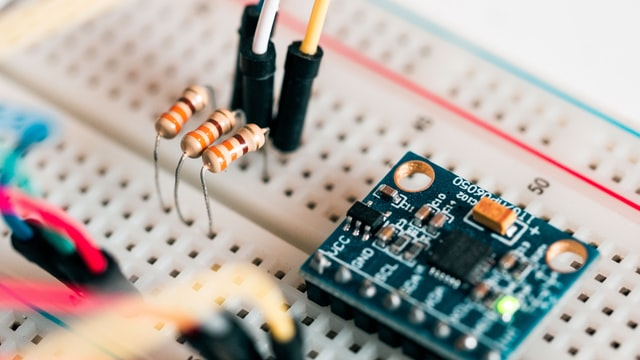# How to use a Multimeter

20 Jan
2022

Learn how to use an inexpensive Multimeter to measure voltage, current, resistance and continuity.

## What is a Multimeter and why do you need one?

A Multimeter is a measuring instrumet that is used to determine electrical properties of a circut. They can be obtained for under \$25 (our one was \$8). A multimeter basically combines three essential features into one small box: a voltmeter, ohmeter and ammeter.

### Use cases

A multimeter allows you to understand what is going on in your electrical circuts. Whenever something is not working as it should, this device will be your friend. For example:

• Is a switch on or off?
• Is a wire broken or conducting electricity?
• How much current is flowing?
• How full are my batteries?

### Getting started

To get going with the meausring device, you need to connect two probes. The black probe is used at the COM port, and the red probe in either one of the other ports.
If you want to measure voltage, resistance or low current (under 10A) you can use the mAVΩ setting. If you want to measure high current (greater than 10A CAREFUL! currents above 100mA are leathal) you can use the 10A plug.

The knob of the meter allows for the selection of sensitivity. Measurements should always first be taken on the highest setting and then reduced until the value can be clearly read off the meter, therefore avoiding blowing the fuse of the meter. If a value of 0.00 is displayed, you are probably on a too high setting. If a vlaue of 1. is displayed, the meter has an overload and you are on a too low setting.
'm' denotes milli (a thousandth) so, 200mA denotes 0.2A.
The values displayed on the meter are the maximum value that can be measured at each of the settings.

## What can a Multimeter measure?

### Voltage (Potential Difference, measured in V)

Voltage is the difference in electrical potential between two points. It is defined as the work needed per unit of charge of 1 Coulomb to move a charge between two points. You can think of voltage as the driving force of the circut which is due to an inbalance of charge at the positive and negative power source electrodes.
1 volt = 1 joule (of work) per 1 coulomb (of charge)

To measure voltage the Multimeter does not conduct electricity. It simply measures the difference in potential between the two points of the probes. The use of the meter for this is simple. The probes are pleased at the two points between which the potential will be meausred, eg. on both poles of a battery.

### Current (measured in A)

Current is the rate (speed) at which electrons are flowing through the electrical circut.

High current is very dangerous and extreme caution must be taken if high currents are tested.

To measure curent, the multimeter conducts and measures the rate at which electrons are flowing through it. Therefore the probes must be placed in series with the circut therefore forcing the electrons to flow through the meter.

### Resistance (Measured in Ω)

Resistance is a measure of the opposition to current flow in an electrical circut. It is measured in Ohms (Ω).

Measuring resistance is very much like measuring voltage. The two probes are placed accros the component(s) you wish to measure the resistance of.

### Continuity

This is a simple, useful setting used to tell you if a circut conducts or not.
If the two probes are connected, the meter beeps. Otherwise, it does not beep.
You can use this to check if wires are broken.Engineering

### How to use a Breadboard

A breadboard is a super useful piece of kit to connect up electrical circuts. This short tutorial explains how it works.

by:Jan 20, 2022